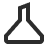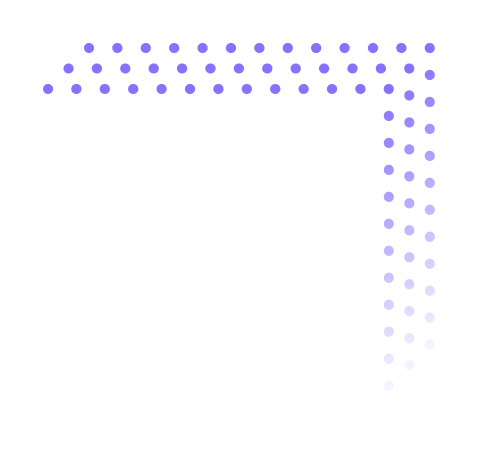# Dataflow Reference

## Data processing building blocks

##### Toric is a visual data experience – from exploration, processing and visualization. Nodes can be connected together to build powerful data-pipelines.#### Breakout

Extracts a set of columns from the input table.#### Clean Up

Trim white spaces, and remove blank rows or non-printable characters in table rows.#### Coalesce

Combine one or many columns based on the first non-empty value.#### Column Rename

Rename one or more columns in a table, modifying the display names.#### Data Tagging

Create a new column and tag data based on a set of rules.#### Diff

Identify changes in data between two source versions.#### Duplicate Column

Select and create a copy of any column in your data.#### Edit Column

Change the column type or name of one or more columns.#### Element At Index

Gives the position (index) of a value in a table.#### Element Index

Outputs the position (index) of a value in a table.#### Filter

Filters data based on the configuration.#### Index Of Element

Provides the index of a specific value (opposite to ElementAtIndex).#### Keep Rows

Keep rows at the top or bottom of a table.Promotes the next row of a table as the new headers and inference types.#### Range Selector

Displays all the rows between two rows indexes.#### Remove Columns

Remove rows from the top or bottom of a table.#### Remove Rows

Remove rows from the top or bottom of a table.#### Reorder Columns

Reorders columns in a table relative to other columns.#### Sort

Sort table records in ascending or descending order based on selected fields.#### Text Truncate

Takes several characters from the left or right side of the text as a new column.#### Unnest

Translate an array of columns into a set of records.#### Append

Append rows from two different tables, sharing columns with the same name and type.#### Combine Columns

Combine columns by merging column values into a single value.#### Concatenate

Concatenates two tables as a single table, merging the columns of the two tables.#### Data Mapper

Remap data from existing fields into a new data structure.#### Find and Replace

Replaces the value of a cell by searching and matching conditions.#### Group By

Groups table records based on the selected fields.#### Join

Combine columns, entire tables, or both by joining them by columns and direction selected.#### Place As Index

Outputs the row at a specific index in a table.#### Pluck Column

Selects and copies a specific column from a table.#### Repeat

Displays appended copies of the input value.#### Split

Splits a column into multiple columns using a delimiter value.#### String Concatenate

Combines two columns together into a new column.#### Summarize

Creates a new table of summarized values, preserving groups.#### Ungroup

Remove record groups by flattening the table.#### Unique

Extracts the distinct values from input data.#### Boolean

Generates a true or false value that is constant.#### Currency

Generates a value of currency respecting the localization settings.#### Date

Generates a Date value that is constant.#### Feet

Generates a unit of measure in feet. Use this node to create a constant value.#### Number

A number that can be changed into a decimal (float), integer, or an unassigned integer.#### Random Number

Produces a random number that regenerates every time the node is evaluated.‍#### Stable Random

Produces a random number that remains the same regardless if the node is re-evaluated.#### Text

An editable text field that can be changed.#### Time Now

A Generate node that shows the Time Now#### Unit

Generates a unit of measurement in high precision.#### Absolute

Converts any negative number into positive number.Add two single numbers or two columns of any number type.#### Average

Calculates the average of numbers in a column.#### Ceiling

Rounds the input number to the highest integer number closest to the input.#### Column Names

Get a list of column names in your data.#### Cosine

A function that provides the length of the adjacent side divided by the hypotenuse length.#### Count

Calculates the number of rows in a table.#### Divide

Divide two single numbers or two columns of any number type.#### Field Calculator

Adds new columns with results calculated from other columns.#### Floor

Rounds the input number to the lowest integer number closest to the input.#### Formula

Type formulas using data fields to calculate new values.#### Group Count

Calculates the number of groups in a table.#### Max

Returns the maximum value of the selected number column in the input.#### Min

Returns the minimum value of the selected number column in the input.#### Multiply

Multiply two single numbers or two columns of any number type.#### Round

Replaces the input number with the nearest integer number.#### Sine

Provides the input number of a ratio between the long side (hypotenuse) and the side that is counter to an acute angle in a right triangle.#### Subtract

Subtract two single numbers or two columns of any number type.#### Sum

Adds all the values in a column with the total value.#### Bigger Than

Output is true if the value of input A is bigger than the value of input B.#### If

Evaluates if the condition of an input value is true or false.#### Is Equal

Output is true if the value of inputA and inputB are the same.#### Less Than

Output is true if the value of inputA is less than the value of inputB.

Coming Soon## Get started today

### Start a trial to access Toric and build a data pipeline in minutes.